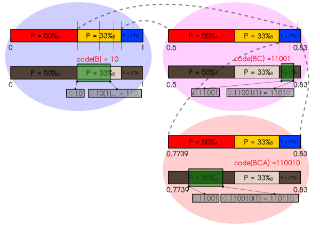# Arithmetic coding

Topic | v1 | created by janarez |Description

Arithmetic coding is a form of entropy encoding used in lossless data compression. Normally, a string of characters such as the words "hello there" is represented using a fixed number of bits per character, as in the ASCII code. When a string is converted to arithmetic encoding, frequently used characters will be stored with fewer bits and not-so-frequently occurring characters will be stored with more bits, resulting in fewer bits used in total. Arithmetic coding differs from other forms of entropy encoding, such as Huffman coding, in that rather than separating the input into component symbols and replacing each with a code, arithmetic coding encodes the entire message into a single number, an arbitrary-precision fraction q where 0.0 ≤ q < 1.0. It represents the current information as a range, defined by two numbers.

##### Relations

In signal processing, data compression, source coding, or bit-rate reduction is the process of encodi...##### Resources

5.0 rating 3.0 level 6.0 clarity 3.0 background – 1 rating

Arithmetic coding is a data compression technique that encodes data (the data string) by creating a c...This introduction to arithmetic coding is divided in two parts. The first explains how and why arit...